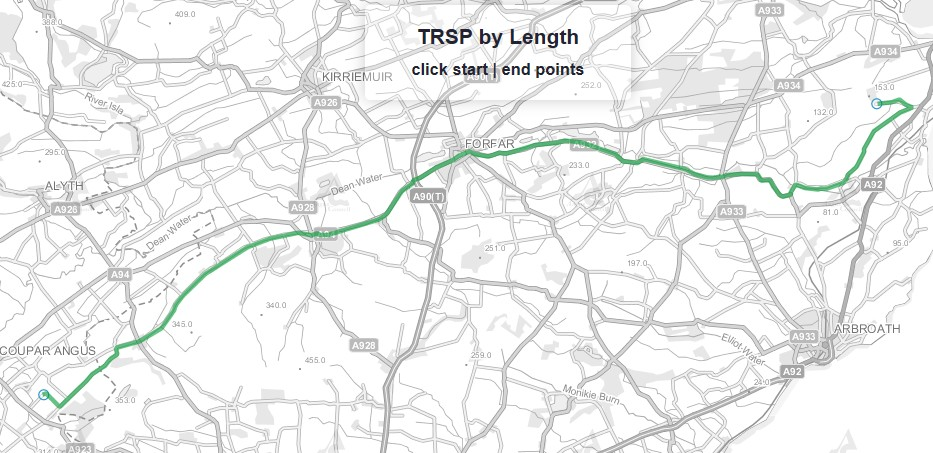# pgRouting TRSP in OpenLayers3

I've managed, after some hacking, to write a wrapper to the pgr_trsp function in pgRouting that allows me to use the Turn Restricted Shortest Path to route between two points on the map. It is in the same structure as the Dijkstra Shortest Path wrapper with just a few minor changes. I wanted/needed to use the TRSP as my ITN network is built using the road routing information (RRI) and turns and restrictions are essential to correct routing across the network topology.

I wrote two functions - one for time costs (quickest journey) and one for distance costs (shortest journey). I created a different layer for each one in Geoserver and a separate HTML/JS file for each function on the web server. One could probably write something that allowed you to pick your algorithm from a list on a single page...

``````-- Function: routing.pgr_fromatob_trsp_length(character varying, double precision, double precision, double precision, double precision)
-- DROP FUNCTION routing.pgr_fromatob_trsp_length(character varying, double precision, double precision, double precision, double precision);

CREATE OR REPLACE FUNCTION routing.pgr_fromatob_trsp_length(IN tbl character varying, IN x1 double precision, IN y1 double precision, IN x2 double precision, IN y2 double precision, OUT seq integer, OUT gid integer, OUT name text, OUT heading double precision, OUT cost double precision, OUT geom geometry)
RETURNS SETOF record AS
\$BODY\$
DECLARE
sql     text;
rec     record;
source	integer;
target	integer;
point	integer;

BEGIN
-- Find nearest node
EXECUTE 'SELECT id::integer FROM itn_network_vertices_pgr
ORDER BY the_geom <-> ST_GeometryFromText(''POINT('
|| x1 || ' ' || y1 || ')'',27700) LIMIT 1' INTO rec;
source := rec.id;

EXECUTE 'SELECT id::integer FROM itn_network_vertices_pgr
ORDER BY the_geom <-> ST_GeometryFromText(''POINT('
|| x2 || ' ' || y2 || ')'',27700) LIMIT 1' INTO rec;
target := rec.id;

-- Shortest path query (TODO: limit extent by BBOX)
seq := 0;
sql := 'SELECT gid, geometry, COALESCE(roadname,dftname) AS name, cost, source, target, ST_Reverse(geometry) AS flip_geom FROM ' ||
'pgr_trsp(''SELECT gid as id, source::int, target::int, '
|| 'cost_len::float AS cost, rcost_len::float AS reverse_cost FROM '
|| quote_ident(tbl) || '''::text' || ', '
|| source || ', '
|| target || ', '
|| 'true, true, '
|| '''SELECT to_cost, teid AS target_id, feid||COALESCE('''',''''||via,'''''''') AS via_path FROM routing.itn_turn_restrictions''::text), '
|| quote_ident(tbl)
|| ' WHERE gid = id2 ORDER BY seq';

-- Remember start point
point := source;

FOR rec IN EXECUTE sql
LOOP
-- Flip geometry (if required)
IF ( point != rec.source ) THEN
rec.geometry := rec.flip_geom;
point := rec.source;
ELSE
point := rec.target;
END IF;

EXECUTE 'SELECT degrees( ST_Azimuth(
ST_StartPoint(''' || rec.geometry::text || '''),
ST_EndPoint(''' || rec.geometry::text || ''') ) )'

-- Return record
seq     := seq + 1;
gid     := rec.gid;
name    := rec.name;
cost    := rec.cost;
geom    := rec.geometry;
RETURN NEXT;
END LOOP;
RETURN;
END;
\$BODY\$
LANGUAGE plpgsql VOLATILE STRICT
COST 100
ROWS 1000;
ALTER FUNCTION routing.pgr_fromatob_trsp_length(character varying, double precision, double precision, double precision, double precision)
OWNER TO postgres;
``````The key bit here is the SQL query in the middle of the function. This runs the TRSP function and returns the turn restricted shortest path using length as the cost. It needs to be in the form:

pgr_trsp(text, integer, integer, boolean, boolean, text)

The SQL that adds the turn restrictions took some working out as I entered Quote Hell and it was some time before I worked out just how many single quotes were needed.

The second function is identical but for the use of the cost_time and rcost_time fields instead.

``````-- Function: routing.pgr_fromatob_trsp_time(character varying, double precision, double precision, double precision, double precision)
-- DROP FUNCTION routing.pgr_fromatob_trsp_time(character varying, double precision, double precision, double precision, double precision);

CREATE OR REPLACE FUNCTION routing.pgr_fromatob_trsp_time(IN tbl character varying, IN x1 double precision, IN y1 double precision, IN x2 double precision, IN y2 double precision, OUT seq integer, OUT gid integer, OUT name text, OUT heading double precision, OUT cost double precision, OUT geom geometry)
RETURNS SETOF record AS
\$BODY\$
DECLARE
sql     text;
rec     record;
source	integer;
target	integer;
point	integer;

BEGIN
-- Find nearest node
EXECUTE 'SELECT id::integer FROM itn_network_vertices_pgr
ORDER BY the_geom <-> ST_GeometryFromText(''POINT('
|| x1 || ' ' || y1 || ')'',27700) LIMIT 1' INTO rec;
source := rec.id;

EXECUTE 'SELECT id::integer FROM itn_network_vertices_pgr
ORDER BY the_geom <-> ST_GeometryFromText(''POINT('
|| x2 || ' ' || y2 || ')'',27700) LIMIT 1' INTO rec;
target := rec.id;

-- Shortest path query (TODO: limit extent by BBOX)
seq := 0;
sql := 'SELECT gid, geometry, COALESCE(roadname,dftname) AS name, cost, source, target, ST_Reverse(geometry) AS flip_geom FROM ' ||
'pgr_trsp(''SELECT gid as id, source::int, target::int, '
|| 'cost_time::float AS cost, rcost_time::float AS reverse_cost FROM '
|| quote_ident(tbl) || '''::text' || ', '
|| source || ', '
|| target || ', '
|| 'true, true, '
|| '''SELECT to_cost, teid AS target_id, feid||COALESCE('''',''''||via,'''''''') AS via_path FROM routing.itn_turn_restrictions''::text), '
|| quote_ident(tbl)
|| ' WHERE gid = id2 ORDER BY seq';

-- Remember start point
point := source;

FOR rec IN EXECUTE sql
LOOP
-- Flip geometry (if required)
IF ( point != rec.source ) THEN
rec.geometry := rec.flip_geom;
point := rec.source;
ELSE
point := rec.target;
END IF;

EXECUTE 'SELECT degrees( ST_Azimuth(
ST_StartPoint(''' || rec.geometry::text || '''),
ST_EndPoint(''' || rec.geometry::text || ''') ) )'

-- Return record
seq     := seq + 1;
gid     := rec.gid;
name    := rec.name;
cost    := rec.cost;
geom    := rec.geometry;
RETURN NEXT;
END LOOP;
RETURN;
END;
\$BODY\$
LANGUAGE plpgsql VOLATILE STRICT
COST 100
ROWS 1000;
ALTER FUNCTION routing.pgr_fromatob_trsp_time(character varying, double precision, double precision, double precision, double precision)
OWNER TO postgres;
``````